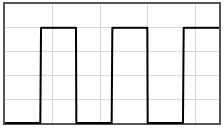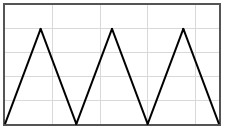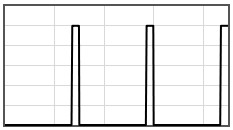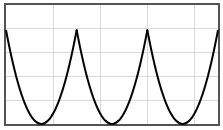PHY.K02UF Molecular and Solid State Physics

## Bloch waves in 1-D

Consider an electron moving in a periodic potential $V(x)$. The period of the potential is $a$, $V(x+a) = V(x)$. The Schrödinger equation for this case is,

$\begin{equation} -\frac{\hbar^2}{2m}\frac{d^2\psi}{dx^2}+V(x)\psi =E\psi . \end{equation}$

Quantum mechanically, the electron moves as a wave through the potential. Due to the diffraction of these waves, there are bands of energies where the electron is allowed to propagate through the potential and bands of energies where no propagating solutions are possible. The Bloch theorem states that the propagating states have the form,

$\begin{equation} \psi = e^{ikx}u_k(x). \end{equation}$

where $k$ is the wavenumber and $u_k(x)$ is a periodic function with periodicity $a$.

There is a left moving Bloch wave $\psi_-=e^{-ikx}u_{k-}$ and a right moving Bloch wave $\psi_+=e^{ikx}u_{k+}$ for every energy. The following form calculates the Bloch waves for a potential $V(x)$ that is specified in the interval between $0$ and $a$. A discussion of the calculation can be found below the form.

 $V(x)$[eV] $x/a$
 The 1-D Potential Energy E = [eV] Mass m = [kg] Lattice constant a  = [m] Potential V(x)  = [eV]
 $\psi_+$ $x/a$ $\psi_-$ $x/a$
 $u_{k+}$ $x/a$ $u_{k-}$ $x/a$ $e^{ik\xi}$ $\xi = x/a$ $e^{-ik\xi}$ $\xi = x/a$ $\psi^*\psi$ $x/a$ $\frac{2ma}{\hbar}v_k$ $x/a$
 $\psi_1$ $\psi_2$ $x/a$ $x/a$

Data:

Standard mathematical functions abs(x), acos(x), asin(x), atan(x), cos(x), exp(x), log(x), pi = 3.141592653589793, pow(x,y) = xy, round(x), sin(x), sqrt(x) tan(x) can be used in the form. In addition, the Heaviside step function H(x) can be used. Multiplication must be specified with a '*' symbol, 3*cos(x) not 3cos(x). Powers are specified with the 'pow' function: x² is pow(x,2) not x^2.

Some potentials that can be pasted into the form are given below.The Schrödinger equation for a particle moving in one dimension is a second order linear differential equation thus any solution can be written in terms of two linearly independent solutions. Although any two linearly independent solutions can be used, only two are eigen functions of the Hamiltonian. Due to the symmetry of the potential, these two are simultaneously eigen functions of the translation operator $\text{T}\psi(x)= \psi(x+a)=\lambda \psi(x)$. Here $\lambda$ is the eigen value of the translation operator $\text{T}$. If periodic boundary conditions are used for a potential with $N$ unit cells, then applying the translation operator $N$ times brings the function back to its original position,

$\begin{equation} \text{T}^N\psi(x)=\psi(x+Na)=\lambda^N\psi(x)=\psi(x). \end{equation}$

The eigenvalues of the translation operator are therefore the solutions to the equation $\lambda^N=1$. These solutions are,

$\begin{equation} \lambda_j=\exp(i2\pi j/N)=\exp(i2\pi aj/L)=\exp(ik_ja), \end{equation}$

where $j$ is an integer between $-N/2$ and $N/2$, $L=Na$ is the length of the crystal, and $k_j=2\pi j/L$ are the allowed $k$-values in the first Brillouin zone.

Notice that a Bloch wave is an eigenfunction of the translation operator with eigenvalue $e^{ika}$,

$\begin{equation} \text{T}e^{ikx}u_k(x)=e^{ik(x+a)}u_k(x+a)=e^{ika}e^{ikx}u_k(x). \end{equation}$

We proceed by arbitrarily choosing two linearly independent solutions and calculating them numerically. Using these solutions, the translation operator is constructed and then the eigen functions of the translation operator are determined. The eigen functions of the translation operator are Bloch waves and are also eigen functions of the Hamiltonian.

For the numerical calculation, it is convenient to measure length in units of $\xi=x/a$. The Schrödinger equation then takes the form,

$\begin{equation} \frac{d^2\psi}{d\xi ^2}+\frac{2ma^2}{\hbar^2}(E-V(\xi ))\psi =0, \end{equation}$

where $V(\xi)=V(\xi +1)$. Two linearly independent solutions can be chosen by specifying their values at $\xi=0$. A convenient choice is,

$\begin{equation} \psi_1(0) = 1,\hspace{0.5cm}\frac{d\psi_1}{d\xi}(0) = 0,\hspace{0.5cm}\psi_2(0) = 0,\hspace{0.5cm}\frac{d\psi_2}{d\xi}(0) = 1. \end{equation}$

Any other solution can be written as a linear combination of $\psi_1(\xi)$ and $\psi_2(\xi)$. In particular, $\psi_1(\xi+1)$ and $\psi_2(\xi+1)$ can be written in terms of $\psi_1(\xi)$ and $\psi_2(\xi)$. These solutions are related to each other by the matrix representation of the translation operator. 

\begin{equation} \left[ \begin{array}{c} \psi_1(\xi+1) \\ \psi_2(\xi+1) \end{array} \right]= \begin{bmatrix} T_{11} & T_{12} \\ T_{21} & T_{22} \end{bmatrix}\left[ \begin{array}{c} \psi_1(\xi) \\ \psi_2(\xi) \end{array} \right]. \end{equation}

The elements of the translation matrix can be determined by evaluating the equation above and its derivative at $\xi=0$.

\begin{equation} \left[ \begin{array}{c} \psi_1(\xi+1) \\ \psi_2(\xi+1) \end{array} \right]= \begin{bmatrix} \psi_1(1) & \frac{d\psi_1}{d\xi}(1) \\ \psi_2(1) & \frac{d\psi_2}{d\xi}(1) \end{bmatrix}\left[ \begin{array}{c} \psi_1(\xi) \\ \psi_2(\xi) \end{array} \right]. \end{equation}

The translation operator is related to the Wronskian of these two linearly independent solutions. The Wronskian is,

\begin{equation} W(\xi)= \begin{vmatrix} \psi_1(\xi) & \frac{d\psi_1}{d\xi}(\xi) \\ \psi_2(\xi) & \frac{d\psi_2}{d\xi}(\xi) \end{vmatrix}. \end{equation}

Using Abel's identity, one can show that for these two solutions,

\begin{equation} W(\xi)= \begin{vmatrix} \psi_1(\xi) & \frac{d\psi_1}{d\xi}(\xi) \\ \psi_2(\xi) & \frac{d\psi_2}{d\xi}(\xi) \end{vmatrix} = \psi_1(\xi)\frac{d\psi_2}{d\xi}(\xi)-\psi_2(\xi)\frac{d\psi_1}{d\xi}(\xi)=1. \end{equation}

The eigen values $\lambda$ are the roots of the equation,

\begin{equation} \begin{vmatrix} \psi_1(1)-\lambda& \frac{d\psi_1}{d\xi}(1) \\ \psi_2(1) & \frac{d\psi_2}{d\xi}(1)-\lambda \end{vmatrix} = \lambda^2 -\left(\psi_1(1)+\frac{d\psi_2}{d\xi}(1)\right)\lambda + \psi_1(1)\frac{d\psi_2}{d\xi}(1)-\psi_2(1)\frac{d\psi_1}{d\xi}(1)=0. \end{equation}

Using the result of Abel's identity yields the equation,

\begin{equation} \lambda^2 -\left(\psi_1(1)+\frac{d\psi_2}{d\xi}(1)\right)\lambda +1=0. \end{equation}

The eigen values are,

\begin{equation} \lambda_{\pm} = \frac{\alpha\pm\sqrt{\alpha^2-4}}{2},\hspace{1cm}\alpha= \left(\psi_1(1)+\frac{d\psi_2}{d\xi}(1)\right). \end{equation}

If $\alpha^2 < 4$, the eigen values are complex and,

\begin{equation} |\lambda_{\pm}|^2=\lambda_{\pm}^*\lambda_{\pm} = \frac{\alpha\mp i\sqrt{4-\alpha^2}}{2}\frac{\alpha\pm i\sqrt{4-\alpha^2}}{2}=1, \end{equation}

the eigen values are on the complex circle. Any number on the complex circle can be written as $e^{i\theta}$ where $\tan \theta = \frac{\text{Im}[\lambda]}{\text{Re}[\lambda]}$. Since the eigen values of the translation operator have the form $e^{ika}$,

\begin{equation} k_+a = \text{atan}\left(\frac{\sqrt{4-\alpha^2}}{\alpha}\right),\hspace{1cm}k_-a = \text{atan}\left(\frac{-\sqrt{4-\alpha^2}}{\alpha}\right)=-k_+a. \end{equation}

If $\alpha > 2$, both eigen values are real and $0 < \lambda_- < 1 < \lambda_+$. If $\alpha < -2$, both eigen values are real and $\lambda_- < -1 < \lambda_+ < 0$. These correspond to exponentially growing or decaying solutions which occur when the energy lies in a forbidden band gap.

The (unnormalized) Bloch waves are,

\begin{equation} \psi_+(\xi)=\left(\lambda_+-\frac{d\psi_2}{d\xi}(1)\right)\psi_1(\xi)+\frac{d\psi_1}{d\xi}(1)\psi_2(\xi), \end{equation} \begin{equation} \psi_-(\xi)=\left(\lambda_--\frac{d\psi_2}{d\xi}(1)\right)\psi_1(\xi)+\frac{d\psi_1}{d\xi}(1)\psi_2(\xi). \end{equation}

The derivatives of the Bloch waves are,

\begin{equation} \frac{d\psi_+}{d\xi}(\xi)=\left(\lambda_+-\frac{d\psi_2}{d\xi}(1)\right)\frac{d\psi_1}{d\xi}(\xi)+\frac{d\psi_1}{d\xi}(1)\frac{\psi_2}{d\xi}(\xi), \end{equation} \begin{equation} \frac{d\psi_-}{d\xi}(\xi)=\left(\lambda_--\frac{d\psi_2}{d\xi}(1)\right)\frac{d\psi_1}{d\xi}(\xi)+\frac{d\psi_1}{d\xi}(1)\frac{\psi_2}{d\xi}(\xi). \end{equation}

The normalized probability current is,

\begin{equation} S=\frac{-i\hbar}{2m}\frac{\left( \psi^* \frac{d\psi}{dx}-\psi \frac{d\psi^*}{dx}\right)}{\int\limits_0^L\psi^*\psi dx}. \end{equation}

Here $L=Na$ is the size of the crystal and $N$ is the number of unit cells in the crystal. The probability current decreases with the size of the crystal because the electron spreads out over the whole crystal. For a Bloch wave, the probability current is constant everywhere in $x$. In the forbidden band gap, the eigen functions of the Hamiltonian are real and thus the probability current is zero.

The velocity associated with a Bloch wave is the probability current divided by the average electron density $1/Na$,

\begin{equation} v_k=\frac{-i\hbar a}{2m}\frac{\left( \psi^* \frac{d\psi}{dx}-\psi \frac{d\psi^*}{dx}\right)}{\int\limits_0^a\psi^*\psi dx}. \end{equation}

Next we show that the velocity associated with a Bloch wave is the same everywhere which must be for a steady state solution so that charge does not build up somewhere. For simplicity, we combine all terms which are not $x$-dependent into a constant $c$.

\begin{align} v_k(x) = c \left( \psi^* \frac{d\psi}{dx} - \psi \frac{d\psi^*}{dx}\right). \end{align}

The derivative with respect to $x$ is,

\begin{align} \nonumber v_k'(x) &= c \left( \frac{d\psi^*}{dx} \frac{d\psi}{dx} + \psi^* \frac{d^2\psi}{dx^2} - \frac{d\psi}{dx} \frac{d\psi^*}{dx} - \psi \frac{d^2\psi^*}{dx^2} \right) \\[1em] &= c\left( \psi^* \frac{d^2\psi}{dx^2} - \psi \frac{d^2\psi^*}{dx^2} \right). \end{align}

From the Schrödinger equation we know,

\begin{equation*} -\frac{\hbar^2}{2m} \frac{d^2\psi}{dx^2} + V(x) \psi = E \psi, \end{equation*} \begin{equation} \frac{d^2\psi}{dx^2} = \frac{2m}{\hbar^2} (V(x) - E) \psi, \end{equation}

and also

\begin{align} \frac{d^2\psi^*}{dx^2} = \frac{2m}{\hbar^2} (V(x) - E) \psi^*, \end{align}

because $E$ and $V(x)$ are real.

Substituting into the expression for $v_k'$ we get,

\begin{align} \nonumber v_k'(x) &= c \left( \psi^* \frac{2m}{\hbar^2} (V(x)-E) \psi - \psi \frac{2m}{\hbar^2} (V(x) - E) \psi^* \right) \\[1em] &= \frac{2mc}{\hbar^2} (V(x)-E) \left( |\psi|^2 - |\psi|^2 \right) = 0 \label{eq:vprime}. \end{align}

By integrating equation \ref{eq:vprime} we find that,

\begin{align} v_k(x) = \text{const.} \end{align}

The rather intuitive result, that $v_{k+}$ (velocity of $\psi_+$) and $v_{k-}$ (of $\psi_-$) have the same absolute value, but opposite signs, can be proven as follows. First, we need to show that $\psi_+$ and $\psi_-$ are a complex conjugate pair. Substituting the Bloch form of the solution for the right going wave ($\psi_+ = e^{ikx} u_{k+}$) into the Schrödinger equation yields,

\begin{equation*} -\frac{\hbar^2}{2m} \frac{d^2\psi_+}{dx^2} + V(x) \psi_+ = E \psi_+ \end{equation*}, \begin{equation} -\frac{\hbar^2}{2m} \frac{d^2(e^{ikx} u_{k+})}{dx^2} + V(x) e^{ikx} u_{k+} = E e^{ikx} u_{k+}. \end{equation}

The complex conjugate of this equation is,

\begin{align} \label{eq:rightgoing_cc} -\frac{\hbar^2}{2m} \frac{d^2(e^{-ikx} u_{k+}^*)}{dx^2} + V(x) e^{-ikx} u_{k+}^* &= E e^{-ikx} u_{k+}^*. \end{align}

But since the Schrödinger equation for the left going wave ($\psi_- = e^{-ikx} u_{k_-}$) is,

\begin{align} \label{eq:leftgoing} -\frac{\hbar^2}{2m} \frac{d^2(e^{-ikx} u_{k-})}{dx^2} + V(x) e^{-ikx} u_{k-} &= E e^{-ikx} u_{k-}, \end{align}

we see that $u_{k+}^* \equiv u_{k-}$ by simply comparing equations \ref{eq:rightgoing_cc} and \ref{eq:leftgoing}, which also means,

\begin{align*} \psi_+^*(x) \equiv \psi_-(x). \end{align*}

From this we can calculate the velocities of the left and right moving waves,

\begin{align*} v_{k+}(x) &= c \left( \psi_+^* \frac{d\psi_+}{dx} - \psi_+ \frac{d\psi_+^*}{dx}\right) \\[.7em] &= c \left( \psi_+^* \frac{d(\psi_+^*)^*}{dx} - (\psi_+^*)^* \frac{d\psi_+^*}{dx}\right) \\[.7em] &= c \left( \psi_- \frac{d\psi_-^*}{dx} - \psi_-^* \frac{d\psi_-}{dx}\right) \\[.7em] &= -c \left( \psi_-^* \frac{d\psi_-}{dx} - \psi_- \frac{d\psi_-^*}{dx} \right) = -v_{k-}(x). \end{align*}

So the velocities of the left and right moving waves are equal in amplitude and opposite in sign.

 W. Magnus and S. Winkler, Hill's Equation, Dover 1966.